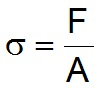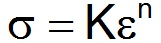Feb 26, 2016

Feb 26, 2016

Feb 26, 2016

# True Stress and Strain

Also, see Engineering Stress and Strain

In the field of materials science and engineering, understanding how materials deform under external forces is crucial for designing and optimizing structural components. Two fundamental concepts used to describe the deformation behavior of materials are true stress and true strain. In this article, we delve into the definitions, significance, and applications of true stress and true strain, shedding light on their role in characterizing material behavior.

## True Stress Definition

True stress is a measure of the applied force per unit area at any given point in a deforming material. Unlike engineering stress, which considers the original cross-sectional area of the specimen, true stress takes into account the instantaneous cross-sectional area that changes as the material undergoes deformation. The formula for calculating true stress is:

True Stress = Force / Instantaneous Cross-Sectional Area

As the material deforms and thins, the true stress increases since the actual area subjected to the force decreases. True stress provides a more accurate representation of the material's resistance to deformation as it accounts for changes in cross-sectional area during the process.

The true stress (ø) uses the instantaneous or actual area of the specimen at any given point, as opposed to the original area used in the engineering values.## True Strain

True strain is a measure of the deformation or elongation experienced by a material relative to its original length. Similar to true stress, true strain considers the instantaneous length of the material rather than the original length. It is expressed as the natural logarithm of the ratio of the final length (l_f) to the original length (l_0):

True Strain = ln(l_f / l_0)

True strain provides a more precise description of the material's elongation, particularly when it undergoes significant deformation. It accounts for changes in length as the material stretches and provides valuable insights into its behavior under external forces.

The true strain (e) is defined as the instantaneous elongation per unit length of the specimen.## Relation between True Stress and True Strain

The relationship between true stress and true strain i.e. the flow curve can be expressed using the power law:where K is called the strength coefficient and n the Strain Hardening exponent.

## Relation between True and Engineering Stress-Strain

For a given value of the load and elongation, the true stress is higher than the Engg. stress, while the true strain is smaller than the Engg. Strain. The relationship between the true and engineering values is given by the formula:## Significance and Applications:

1. Material Characterization: True stress and true strain play a crucial role in characterizing the mechanical properties of materials. By plotting stress-strain curves using true stress and true strain values, engineers and researchers can analyze the material's behavior, including its yield strength, ductility, and ultimate tensile strength. These properties are essential for designing structures and predicting the material's performance in real-world applications.

2. Forming and Manufacturing Processes: In industries such as metalworking and plastic deformation, understanding true stress and true strain is vital for optimizing forming and manufacturing processes. These processes involve subjecting materials to external forces and deformations, and accurate predictions of their behavior are necessary to ensure product quality and minimize waste.

3. Finite Element Analysis: True stress and true strain data are utilized in computational simulations, such as finite element analysis (FEA), to model and predict the behavior of materials under various loading conditions. FEA helps engineers assess the structural integrity, performance, and durability of components, enabling them to make informed design decisions.

4. Material Testing and Research: In laboratories and research facilities, true stress and true strain measurements are obtained through specialized equipment, such as tensile testing machines. These measurements are used to validate theoretical models, study material behavior under different conditions, and develop new materials with improved properties.

## Conclusion:

True stress and true strain are fundamental concepts in understanding material deformation behavior. By considering the changing cross-sectional area and instantaneous length during deformation, true stress and true strain provide accurate representations of a material's response to external forces. Their applications in material characterization, manufacturing processes, computational simulations, and research make them essential tools for engineers and researchers seeking to optimize designs, improve performance, and develop new materials.